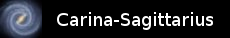So far all of the tasks we have looked at perform simple repeatable sequences of actions. The actions in a task are always repeated unless the task is explicitly terminated. The simple actions described in C++ can of course use any of the control structures that C++ provides, e.g. if, while etc. The only constraint is that simple actions should be relatively short, even if they contain loops, so that they do not monopolise the processor.

The intention is that all complex operations should be described by C++ functions which are invoked as action functions, wait conditions and communication sources and sinks. However, sometimes it is necessary to make the sequence of actions which define a task conditional as well. The multitasking library supports this in a deliberately simple way with an action called “when”. This is specified in terms of a condition function with no parameters which returns a boolean value, just as in the case of the wait action. The behaviour of the when action is as follows. If the condition function returns true when evaluated, the following action is executed. If the condition function returns false, the following action is skipped. This is similar to “`if (condition) action`()” in C++.

The following example demonstrates how the when action is used. This simple task, which behaves rather like a write once cache, will receive a data value from its input once and then send it on its output repeatedly. To emphasise that the receive_from action is controlled by the when action, it is written on the same line, however this makes no difference to the execution of the task.

int  cache_data;
bool have_data = false;

bool no_data()
{
return !have_data;
}

void store_data(int value)
{
cache_data = value;
have_data  = true;
}

int get_data()
{
return cache_data;
}

void write_once_cache(byte input, byte output)
{
send_on(output, get_data);
}

A further conditional action behaves in a similar way to “`while (condition) action()`” in C++. The repeat action also takes a condition function and repeats the action which follows it as long as the condition returns true. If the condition returns false the first time it is evaluated, the following action will not be executed at all. Note: It would be more logical to call this action “while”, but “while” is a reserved word in C++.

Here is an example task called filter which filters out unwanted negative and zero values from its input channel and sends the non-zero, positive values on its output channel.

int  filter_data = 0;

bool unwanted()
{
return filter_data <= 0;
}

void store_data(int value)
{
filter_data = value;
}

int get_data()
{
int data    = filter_data;
filter_data = 0;
return data;
}

void filter(byte input, byte output)
{